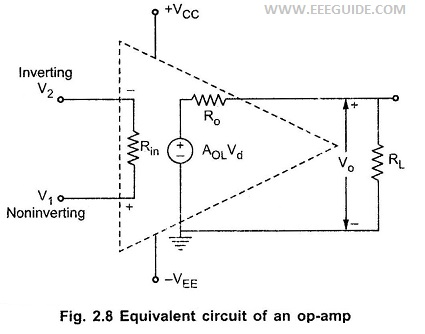## Equivalent Circuit of Practical Op Amp:

The Circuit which represents op-amp parameters in terms of physical components, for the analysis purpose is called equivalent circuit of an op-amp. The Equivalent Circuit of Practical Op Amp is shown in the Fig. 2.8.The Equivalent Circuit of Practical Op Amp shows the op-amp parameters like input resistance, output resistance, the open loop voltage gain in terms of circuit components like Rin, Ro etc. The op-amp amplifies the difference between the two input voltages.

Vo = AOL Vd = AOL (V1 – V2)

where

• AOL = Large signal open loop voltage gain.
• Vd = Difference voltage V1 – V2
• V1 = Noninverting input voltage with respect to ground
• V2 = Inverting input voltage with respect to ground
• Ri = Input resistance of op-amp
• Ro = Output resistance of op-amp

The output voltage is directly proportional to the difference voltage Vd.

It is to be noted that the op-amp amplifies difference voltage and not the individual input voltages. Thus the output polarity gets decided by the polarity of the difference voltage Vd.

The voltage source AOL Vd is the Thevenin’s equivalent voltage source while Ro is the Thevenin’s equivalent resistance looking back into the output terminals.

The equivalent circuit plays an important role in analyzing various op-amp applications as well as in studying the effects of feedback on the performance of op-amp.

Scroll to Top